# How LSTMs solve the problem of Vanishing Gradients?

This article covers the content discussed in the Vanishing and Exploding Gradients and LSTMs module of the Deep Learning course offered on the website: https://padhai.onefourthlabs.in

We discussed in case of RNNs that while back-propagating, gradients might vanish or gradients might explode as discussed in this article and so from there, we move on to the concepts of LSTMs and GRUs(discussed in this article) which uses selective read, write and forget to pass on the relevant information to the state vector.

The gates regulate/control the flow of the information in LSTMs.

Intuition: How gates help to solve the problem of vanishing gradients

During forward propagation, gates control the flow of the information. They prevent any irrelevant information from being written to the state.

Similarly, during backward propagation, they control the flow of the gradients. It is easy to see that during the backward pass, gradients will get multiplied by the gate.

Let’s consider the following output gate:

We can write the hidden state ht as:

ht = (st)*(ot) (Equation 1)

Now let’s say we wish to compute the derivative of the Loss function with respect to W, now in that derivative, at some point we would encounter the derivative of this (ht) with respect to (st) (as we would be back-propagating and ht is derived as the multiplication of st with some numbers(st is multiplied with the ot gate)). And this derivative of (ht) with respect to (st) would just be (ot) as is clear from equation 1. So, that means somewhere in the chain of derivative, (while computing the derivative of the Loss function with respect to W) we would have multiplication with this (ot).

And the same would be there for the other gates as well; somewhere in the chain of the derivative, these gates would be there.

Just as in the forward propagation, things are being multiplied by gates and these gates decides in a way how much of information it needs to pass, during backward propagation also, the gradients are getting multiplied by gates and these gates control the backward flow of information because this gradient is deciding how much of this gradient needs to flow back.

Let say we write s(t-1) as s1 and st as s2(that is ‘t’ is 2 in this case).

If we say that s1 did not contribute much to the state s2 that means the value of the output gate(o(t-1)) and the forget gate(ft) would be close to 0 as these two gates are used with s(t-1)(s1 in this case) as shown in the image below(highlighted in red box in the below image):

So, if both the gates i.e the output gate o(t-1) and the forget gate ft are close to 0, then we can say that s(t-1)(s1 in this case) did not contribute much to st(s2 in this case).

Now what would happen in this case during backpropagation is that, we would again have these gates ft and o(t-1) because that would show up somewhere in the chain rule, and since these two quantities(ft and o(t-1)) are close to 0, the overall gradient would go to 0 and we would have a vanishing gradient problem.

The key difference from the vanilla RNN is that the flow of information and the gradients both are controlled by the gates; what this means is that since in this case, we have both ft and s(t-1) close to 0, that means during the forward pass itself, s1 did not contribute anything to s2, so holding s1 responsible for errors in the Loss function does not make sense as the s1 information was blocked by these gates and it did not carry on forward hence the gradients did not flow back. So, this synchronous thing that whatever happens in the forward pass also happens in the backwards pass; because the gates did not allow the information to flow forward hence the information is not flowing backward, this kind of vanishing problem is okay, it does not the effect the model, if we did not contribute in the forward pass then we don’t need any feedback in the backward pass.

Revisiting RNNs:

Let’s say we want to compute the gradient of the Loss function with respect to W, so it would be summation of derivative of loss function with respect to W over all possible paths as discussed in previous articles, and since this summation over all the paths is there, for the overall gradient to vanish, gradients along all these paths must vanish and the overall gradient would explode if gradient along any one of the path explodes.

Dependency Diagram for LSTMs

The equations involved in the LSTM are as follows: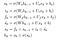First 3 equations are of the gates used in LSTMs, then the next 3 equations represent the states that we compute and last two equations(for sk and hk) compute/represent the output for the LSTM

We have two inputs here(in the above equations), h(k-1) and s(k-1) to compute the new state sk and hk, sk(~) is just an intermediate input(temporary state) and not an actual input and is computed from h(k-1). xk is also there but we are not going to consider it here just like in the case of dependency diagram of RNN where we don’t consider the input x and show all the computations in the terms of state only.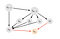This is the dependency diagram to go from one state(s(k-1), h(k-1)) to another state(sk, hk in this case) in case of LSTM.

The same thing is going to repeat at subsequent time steps also meaning that the dependency diagram for a particular time step looks exactly the same as that at some other time step with the only change being in the subscript of the state vector i.e at 1st time step it would be s1, h1; at 2nd time step it would be s2, h2 and so on.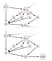ht represents the final state at time step t from which we compute the Loss value.

We will focus on one of the weight and the same argument will hold for other weights also. Let’s consider weight Wf(in red in the below image).

We are interested in knowing if the gradient flows to Wf through sk(we are considering the scenario just like in case of RNNs i.e if Wf was wrong hence sk was wrong and hence the loss was high then we need to pass this information back to Wf through sk).

It is enough to show that the gradient flows up to sk because from sk, Wf is very nearby(from sk) and if the gradient flows up to sk then it’s okay. To reach up to sk, multiple time steps are there and from sk to Wf only two more steps are there so we need to just ensure that gradient flows up to sk.

Now from L(theta) to sk, we have multiple paths and for the case of vanishing gradients, we need to show that the gradient does not vanish along any one of these paths.

As there are multiple paths, the overall gradient of L(theta) with respect to sk is going to be the sum of derivative across all these paths. And now to show that the overall gradient does not vanish, it would be sufficient to show that gradient does not vanish across one of these paths.

So, we have taken one such path(highlighted in blue nodes in the below image) and let’s say we call the gradient across that path as t0. So, this t0 would look like:

We will not bother much about the highlighted part(in the image below) as this is directly connected to ht and along a single path the gradient may not vanish so that’s okay for us. The derivative of ht with respect to st would also be okay, our main concern is with the part in the blue in the image below as that part would again be a multiplication of multiple terms.

We have ht as the following:

So, ht is given the output gate multiplied with the sigmoid over st

Every element of ht depends only on one element of ot and one element of st, so we would have:

ht1 = (ot1)*(sigmoid of st1)

Now ht is a ‘d’ dimensional vector and st is also a ‘d’ dimensional vector, so the derivative of ht with respect to st is going to be a ‘d X d’ dimensional matrix with all the off-diagonal elements as 0(as discussed in the Vanishing and Exploding Gradients article: https://medium.com/@prvnk10/vanishing-and-exploding-gradients-52af750ede32). So, this matrix would be a diagonal matrix. The first element of this matrix would be:

So, we can write the derivative of ht with respect to st as: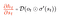Here, D means it is a Diagonal matrix with diagonal elements as the Hadamard product of ot with the derivative of sigmoid of corresponding st value.

Now we want to compute the next term in the equation of t0.

So, we consider the derivative of st with respect to s(t-1).

We have st as the following:

Now this st(~) also depends on s(t-1) as is clear in the below image

The derivative of st with respect to s(t-1) is going to be the sum of the derivative of two terms(as in the formula of the st) with respect of s(t-1) and our goal is show that the overall gradient does not vanish. So, we make an assumption that let’s say derivative of st(~) with respect to s(t-1) actually vanishes and we show that the derivative of the first term(i.e ( ft * s(t-1) ) ) with respect to s(t-1) does not vanish then our work would be done as some value would be there which would imply that the gradient does not vanish. So, we only focus on the first quantity(highlighted in the below image).

Now we have st as:

So, we can say that every element of st depends on only one element of ft and one element of s(t-1). And st is a ‘d’ dimensional vector and s(t-1) is also a ‘d’ dimensional vector, so the derivative of st with respect to s(t-1) would be a matrix with dimensions ‘dX d’ and with off-diagonal elements as 0.

Similarly, we can write the derivative of state at some other time step as well with respect to the previous state as a diagonal matrix with off-diagonal elements as 0 and the diagonal elements as the value of the forget gate at the corresponding index.

So, the overall term t0 looks as:

Now if we have the multiplication of multiple diagonal matrices, we can write the resultant as a single diagonal matrix with corresponding elements of all the matrices being multiplied. Example:

If we multiply many diagonal matrices then the product is a product of all the corresponding diagonal elements(so we get a matrix whose elements is the product of all the corresponding diagonal elements)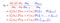In the last equation, we have written the single diagonal matrix(in blue) as the product of all the forget gates from time step k+1 to time step t.

Now the gradients would vanish if all the forget gates i.e f1, f2, f3, ….., all the way up to the last forget gate ft are very very small, then, in that case, the entire gradient would vanish so it looks like the gradients could still vanish but the catch here is that if f1 was very small that means s1 did not contribute much to s2(as explained in the start of this article); if f2 is small it means s2 did not contribute much to s3; in turn what it means is that s1 anyways did not contribute much to s2, s2 has also not contributed much to s3 that means s1 did not contribute almost anything to s3, it contributed even less; now if f4 is also small that means s3 did not contribute anything to s4 and hence the same argument that s1 is contributing even less to s4 so based on this we can say that the contribution has vanished by the time we have reached s4 because the gates were very small; in the same way in the backward direction the gradients from s4 will vanish by the time we reach s1 but this kind of vanishing is not a problem because if in the forward direction it did not contribute that in the backward if the gradients do not reach to that state that is okay as no feedback is required in the backward time when the state did not contribute in the forward pass. So, the gates control the flow of information in both directions.

For the overall gradient to explode, we need to show the gradient explodes at least along one of the paths and that would be sufficient to say that the overall gradient explodes.

So, we are considering the derivative of the Loss function with respect to s(k-1) (one of the terms that appear in the chain rule) and we have considered one of the possible paths(in blue nodes in the below image) that lead from Loss value(L) to s(k-1)

The derivative as per the chain rule would look like:

The term in blue parentheses(above image)(3 nodes ht, ot, h(t-1)) would keep repeating all the way back.

Now the derivative of ht with respect to ot is going to a be diagonal matrix as discussed in the case of vanishing gradients(that the derivative of a ‘d’ dimensional vector with respect to a ‘d’ dimensional vector is going to be a ‘d X d’ dimensional matrix).

We have ot(represented as ok for k’th time step) as the following:

Let’s ignore the sigmoid for a moment(anyways it’s just going to add one more term in the chain nothing more than that), then the last two terms in the above equation are constant and the derivative of ok with respect to h(k-1) would simply be Wo.

So, we can write the derivative as: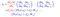All the individual terms in the blue parentheses would have one diagonal matrix and Wo

All the individual terms in the blue parentheses would have one diagonal matrix and Wo, so we expand it out(this is a matrix multiplication), so if we club all the diagonal matrices together we get one large diagonal matrix and Wo is getting multiplied as many times as the number of terms in the chain, we call the magnitude of this large matrix as K, so we have:

So, if the highlighted value in the below image is large then the gradients would explode. So, LSTMs actually could not solve the problem of exploding gradients, the overall gradient could still explode and in practice the way we deal with it is that a gradient has some magnitude and a direction, so we want to go in the direction of the gradient but not with large magnitude so we move in the direction of the gradient but with a small magnitude and the technique is termed as clipping. So, clipping just rescales the gradient so it lies in a certain magnitude range and we can still use the direction of the gradient.

So, while backpropagating if the norm of the gradient exceeds a certain value, it is scaled to keep its norm within an acceptable threshold.

So, in essence, we can say that LSTMs does not have the problem of vanishing gradients(gradients could vanish in case of LSTMs but that would be the case when the information does not flow in the forward direction in the forward pass and that would be okay as discussed in this article).

LSTMs does not actually solve the problem of exploding gradients. Gradients could still explode and the way we deal is that we move in the direction of the Gradient to update the parameters but we move with a small magnitude.